# Count Total Words in a Cell – Excel & Google Sheets

This tutorial will teach you how to count words in a cell in Excel and Google Sheets.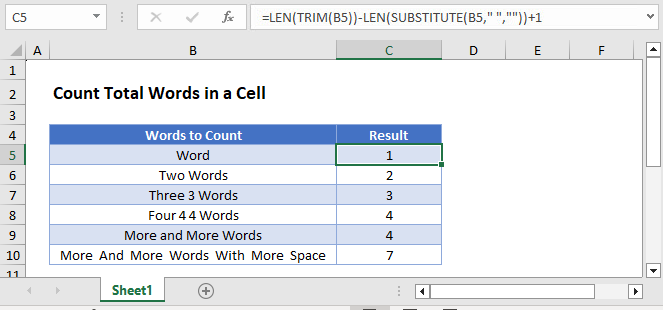## Counting Words

There is no built-in Excel function to count the number of words in a cell. However, we can count the number of spaces, which can tell us how many words are in a cell:

``=LEN(TRIM(B3))-LEN(SUBSTITUTE(B3," ",""))+1``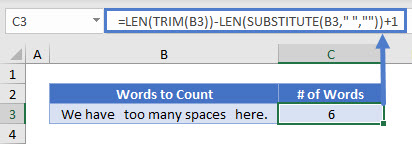Let’s see how this formula works.

## Remove All Spaces

First, we use SUBSTITUTE Function to remove all spaces from the text string.

``=SUBSTITUTE(B3," ","")``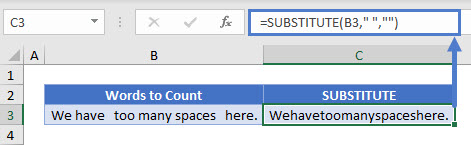Then we determine the length of this space-free string with the LEN Function.

``=LEN(C3) or =LEN(SUBSTITUTE(B3," ",""))``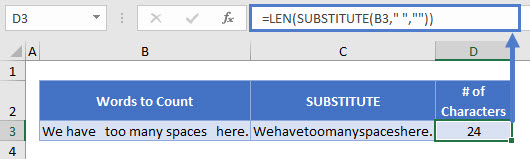We will compare the string length before and after removing the spaces, to count how many spaces the cell contains.

### Remove Extra Spaces

Next, we want to express this string as we normally would (i.e. containing only single spaces between words). The TRIM function removes extra spaces from a text string, leaving a single spaces between each word, so all leading, trailing, and repeated spaces will be excluded.

``=TRIM(B3)``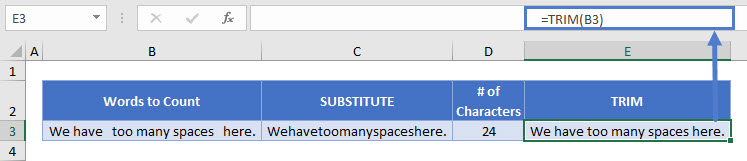Then we determine the length of this normally-arranged text with the LEN function.

``=LEN(E3) or =LEN(TRIM(B3))``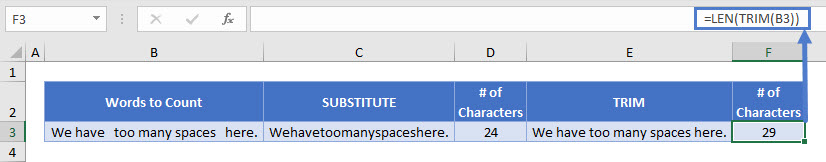Finding the different between the two lengths (with and without spaces) and adding one (since there is no space after the last word) gives us the word count of the cell:

``=F3-D3+1``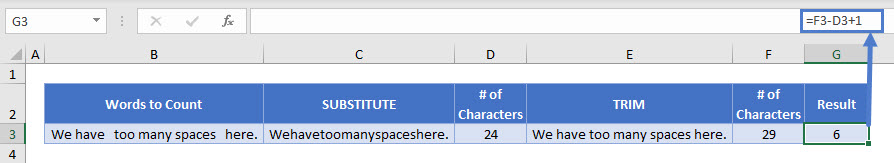Combining these steps gives us the formula:

``=LEN(TRIM(B3))-LEN(SUBSTITUTE(B3," ",""))+1``## Google Sheets –Count Total Words in a Cell

All of the above examples work exactly the same in Google Sheets as in Excel.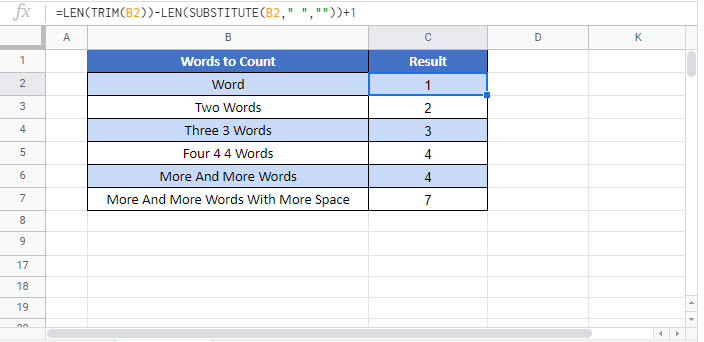### Excel Practice Worksheet

Practice Excel functions and formulas with our 100% free practice worksheets!